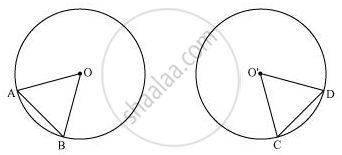# Recall that Two Circles Are Congruent If They Have the Same Radii. Prove that Equal Chords of Congruent Circles Subtend Equal Angles at Their Centres. - Mathematics

Recall that two circles are congruent if they have the same radii. Prove that equal chords of congruent circles subtend equal angles at their centres.

#### Solution

A circle is a collection of points which are equidistant from a fixed point. This fixed point is called as the centre of the circle and this equal distance is called as radius of the circle. And thus, the shape of a circle depends on its radius. Therefore, it can be observed that if we try to superimpose two circles of equal radius, then both circles will cover each other. Therefore, two circles are congruent if they have equal radius.

Consider two congruent circles having centre O and O' and two chords AB and CD of equal lengths.In ΔAOB and ΔCO'D,

AB = CD (Chords of same length)

OA = O'C (Radii of congruent circles)

OB = O'D (Radii of congruent circles)

∴ ΔAOB ≅ ΔCO'D (SSS congruence rule)

⇒ ∠AOB = ∠CO'D (By CPCT)

Hence, equal chords of congruent circles subtend equal angles at their centres.

Concept: Angle Subtended by a Chord at a Point
Is there an error in this question or solution?

#### APPEARS IN

NCERT Class 9 Maths
Chapter 10 Circles
Exercise 10.2 | Q 1 | Page 173